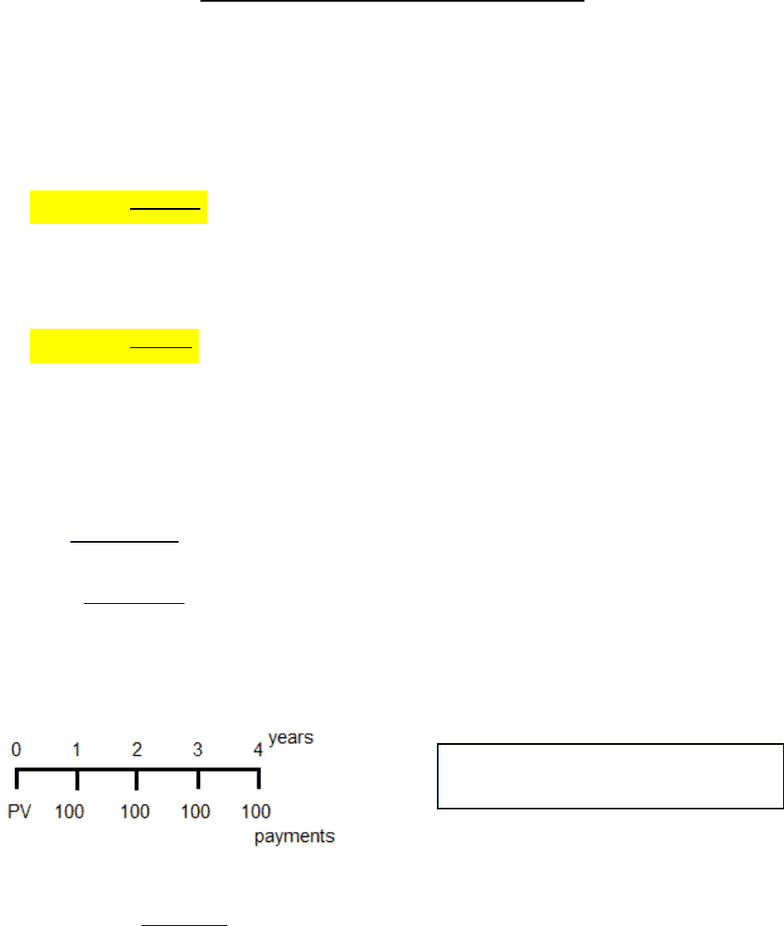Class Notes (1,100,000)
CA (630,000)
UTSC (30,000)
Mathematics (1,000)
MATA32H3 (200)
Lecture 5

# MATA32H3 Lecture Notes - Lecture 5: Integral, Differential CalculusPremium

Department
Mathematics
Course Code
MATA32H3
Professor
Raymond Grinnell
Lecture
5

This preview shows half of the first page. to view the full 3 pages of the document.MATA 32 Lecture 5 Annuities and Limits
Annuities:
Annuities are a sequence of payments of a fixed amount made at regular intervals of
time
Ordinary annuity: Paid at end of intervals
Annuity due: Paid at start of intervals
Formulas for the ordinary annuity (i.e. FV and PV) can be modified for annuity due

o payment amount
o interest per payment period
o total number of payments in the compounding sequence
of an ordinary annuity = ( of all ordinary payments)

of an ordinary annuity = ( of all ordinary payments)
Example:
At the end of each of 4 years, we pay \$100 () into an ordinary annuity and interest is 9% APR.
What is the present value (PV) of the annuity?




How to Interpret PV = \$323.97:
Year 1: 
 ; Payment #1
; Instant amount after payment #1 is made
PV: amount of money needed to invest
in order to issue future payments